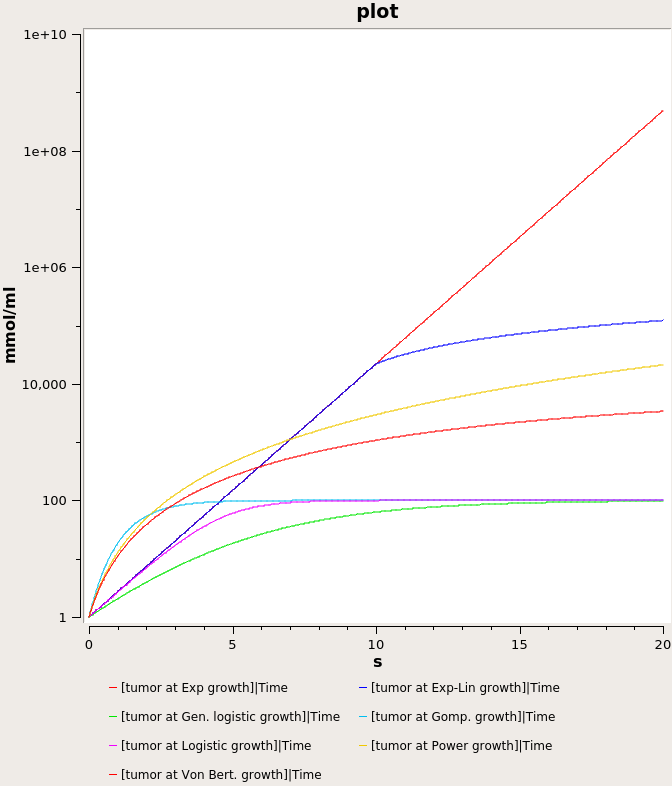## West2019 - Cellular interactions constrain tumor growthModel Identifier
BIOMD0000000820
Short description
These selections of models are described in the paper: Cellular interactions constrain tumor growth by Jeffrey West and Paul K. Newton Significance: A mathematical model relating tumor heterogeneity at the cellular level to tumor growth at the macroscopic level is described based on a statistical mechanics framework. The model takes into account the number of accessible states available to each cell as well as their long-range coupling (population cooperation) to other cells. We show that the degree to which cell populations cooperate determines the number of independent cell states, which in turn dictates the macroscopic (volumetric) growth law. It follows that targeting cell-to-cell interactions or functional coupling among cell populations could be a way of mitigating and controlling tumor growth. Abstract: A tumor is made up of a heterogeneous collection of cell types, all competing on a fitness landscape mediated by microenvironmental conditions that dictate their interactions. Despite the fact that much is known about cell signaling, cellular cooperation, and the functional constraints that affect cellular behavior, the specifics of how these constraints (and the range over which they act) affect the macroscopic tumor growth laws that govern total volume, mass, and carrying capacity remain poorly understood. We develop a statistical mechanics approach that focuses on the total number of possible states each cell can occupy and show how different assumptions on correlations of these states give rise to the many different macroscopic tumor growth laws used in the literature. Although it is widely understood that molecular and cellular heterogeneity within a tumor is a driver of growth, here we emphasize that focusing on the functional coupling of states at the cellular level is what determines macroscopic growth characteristics.
Format
SBML (L2V4)
Related Publication
• Cellular interactions constrain tumor growth.• West J, Newton PK
• Proceedings of the National Academy of Sciences of the United States of America , 2/ 2019 , Volume 116 , Issue 6 , pages: 1918-1923 , PubMed ID: 30674661
• Integrated Mathematical Oncology, Moffitt Cancer Center, Tampa, FL 33612; jeffrey.west@moffitt.org.
• A tumor is made up of a heterogeneous collection of cell types, all competing on a fitness landscape mediated by microenvironmental conditions that dictate their interactions. Despite the fact that much is known about cell signaling, cellular cooperation, and the functional constraints that affect cellular behavior, the specifics of how these constraints (and the range over which they act) affect the macroscopic tumor growth laws that govern total volume, mass, and carrying capacity remain poorly understood. We develop a statistical mechanics approach that focuses on the total number of possible states each cell can occupy and show how different assumptions on correlations of these states give rise to the many different macroscopic tumor growth laws used in the literature. Although it is widely understood that molecular and cellular heterogeneity within a tumor is a driver of growth, here we emphasize that focusing on the functional coupling of states at the cellular level is what determines macroscopic growth characteristics.
Contributors
Submitter of the first revision: Szeyi Ng
Submitter of this revision: Szeyi Ng
Modellers: Szeyi Ng

is (2 statements)
BioModels Database BIOMD0000000820
BioModels Database MODEL1909240001

isDescribedBy (1 statement)
PubMed 30674661

hasProperty (5 statements)
Experimental Factor Ontology cancer
Mathematical Modelling Ontology Ordinary differential equation model
NCIt Heterogeneity
NCIt Cancer Cell Growth Regulation
Systems Biology Ontology rate law

Curation status
Curated

Modelling approach(es)

Tags

#### Connected external resources

SBGN view in Newt Editor

Name Description Size Actions

### Model files

West2019 - Cellular interactions constrain tumor growth.xml SBML L2V4 file for the model 48.30 KB Preview | Download

Fig 2.png PNG plot of the model simulation Figure 2 33.69 KB Preview | Download
West2019 - Cellular interactions constrain tumor growth.cps COPASI 4.24 (Build 197) file for the model 69.15 KB Preview | Download
• Model originally submitted by : Szeyi Ng
• Submitted: Sep 24, 2019 11:06:27 AM
##### Revisions
Legends
: Variable used inside SBML models

Species
Species Initial Concentration/Amount
tumor at Gomp growth

Tumor Size
1.0 mmol
tumor at Von Bert growth

Tumor Size
1.0 mmol
tumor at Power growth

Tumor Size ; power-law modular rate law
1.0 mmol
tumor at Exp growth

Tumor Size
1.0 mmol
tumor at Logistic growth

Tumor Size
1.0 mmol
tumor at Exp Lin growth

Tumor Size
1.0 mmol
tumor at Gen logistic growth

Tumor Size
1.0 mmol
Reactions
Reactions Rate Parameters
=> tumor_at_Gomp__growth compartment*(alpha*tumor_at_Gomp__growth*ln(K)-alpha*tumor_at_Gomp__growth*ln(tumor_at_Gomp__growth)) K = 100.0; alpha = 1.0
=> tumor_at_Von_Bert__growth compartment*(a*tumor_at_Von_Bert__growth^gamma-b*tumor_at_Von_Bert__growth) gamma = 0.666666666666667; a = 4.0; b = 0.2
=> tumor_at_Power_growth compartment*a*tumor_at_Power_growth^gamma gamma = 0.666666666666667; a = 4.0
=> tumor_at_Exp_growth compartment*alpha_0*tumor_at_Exp_growth alpha_0 = 1.0
=> tumor_at_Logistic_growth compartment*alpha*tumor_at_Logistic_growth*(1-tumor_at_Logistic_growth/K) K = 100.0; alpha = 1.0
=> tumor_at_Exp_Lin_growth compartment*(alpha_0_variable*tumor_at_Exp_Lin_growth+alpha_1_variable) alpha_1_variable = 0.0; alpha_0_variable = 1.0
=> tumor_at_Gen__logistic_growth compartment*alpha*tumor_at_Gen__logistic_growth*(1-(tumor_at_Gen__logistic_growth/K)^nu) K = 100.0; nu = 0.3; alpha = 1.0Curator's comment:
(added: 24 Sep 2019, 11:05:47, updated: 24 Sep 2019, 11:05:47)
This figure is reproduced using the COPASI file attached using COPASI 4.24(Build197) by setting time=20 seconds, and take logarithm of Y.